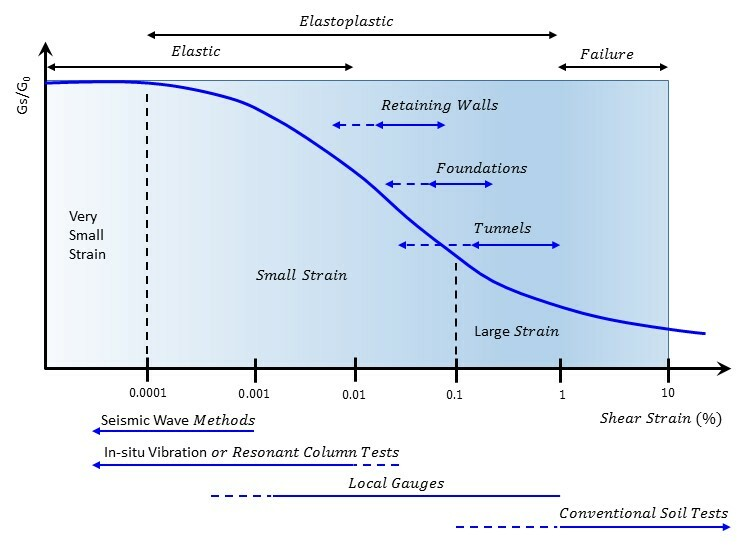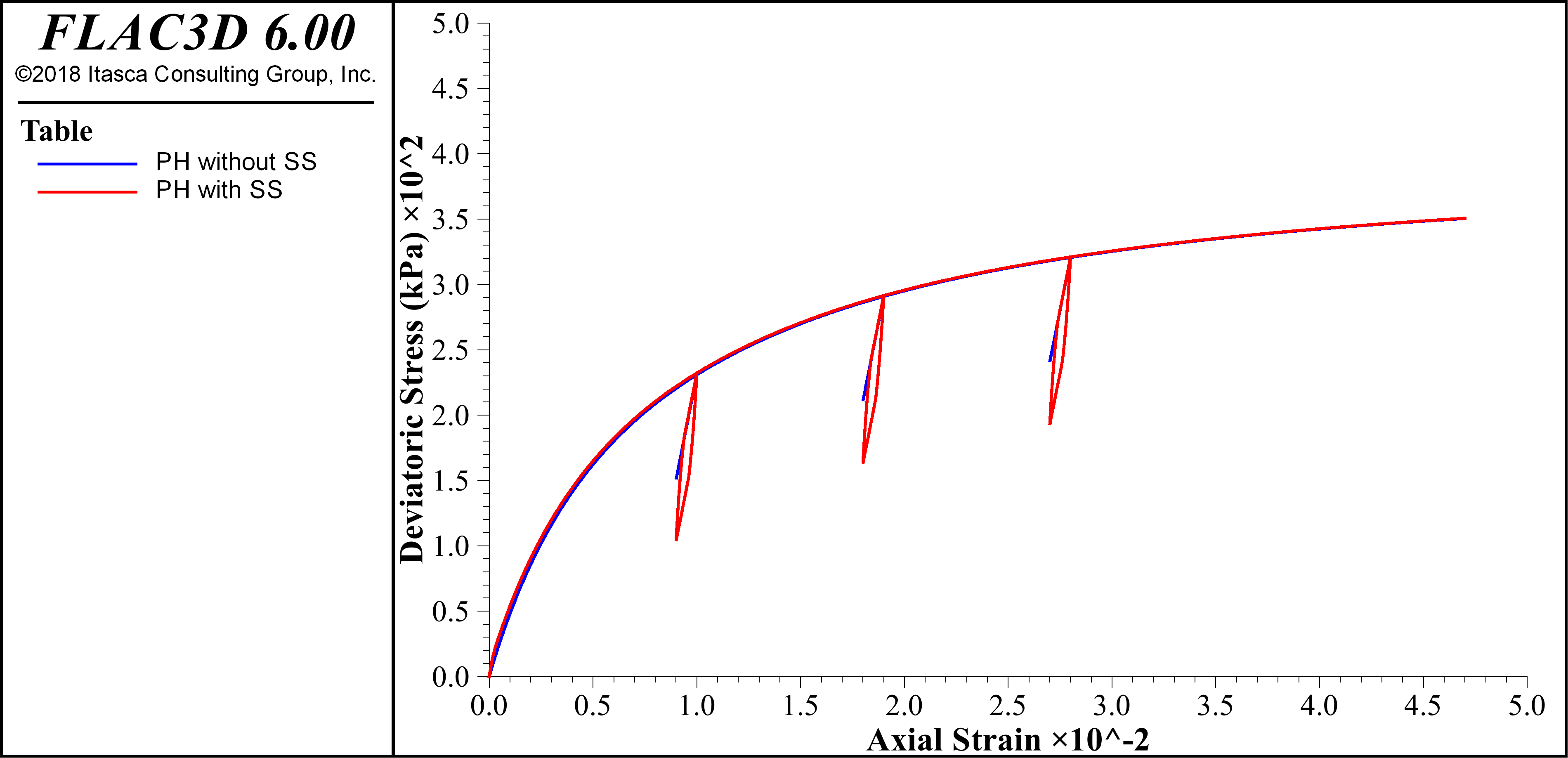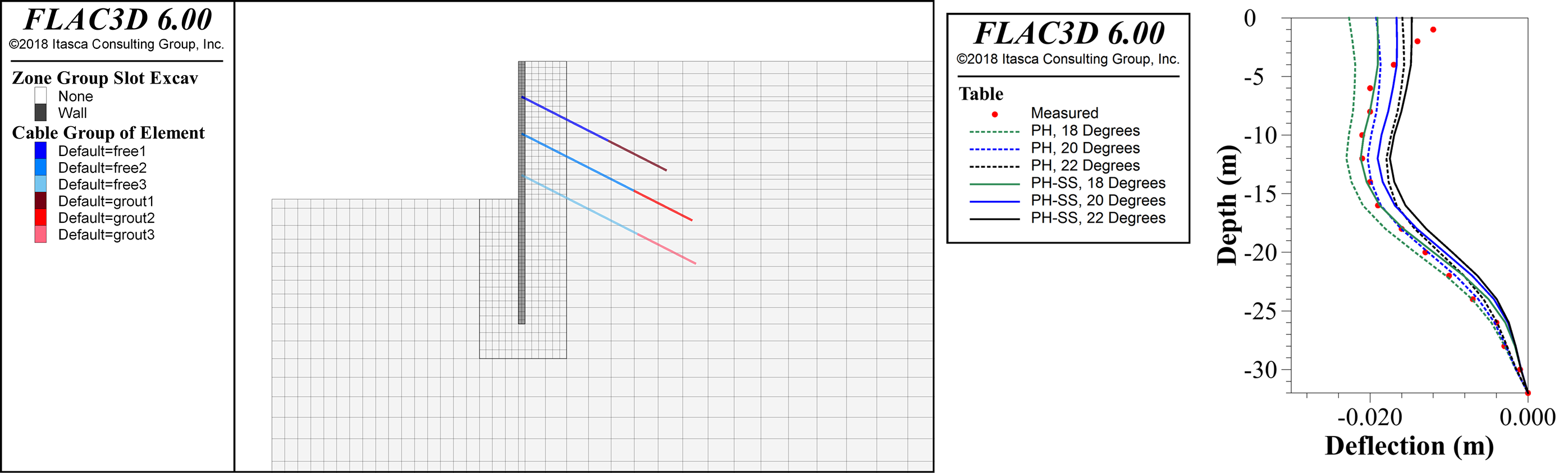## FLAC 3D PH Model with Small-Strain Stiffness

### Introduction

Using the Plastic-Hardening model to simulate unloading-reloading paths, the reloading path will be the reverse of the unloading path. However, this elastic behavior assumes that strains are in the very small strain range. Experiments illustrate that soil stiffness modulus is strain-dependent — it decreases nonlinearly with the increase of shear strain. To take soil strain-dependency into account, a PH small-strain (PHSS) stiffness modulus formulation is now available for the Plastic Hardening model.

Strain can be categorized into three levels: the very small strain, where the stiffness modulus is assumed constant (G0 or sometimes called Gmax); the small strain, where the stiffness modulus reduces nonlinearly as the strain increases; and the large strain, where the soil is in or close to failure and the stiffness modulus is relatively small.

The following figure illustrates. The normalized stiffness modulus degradation curve is shown. It is scaled against geotechnical applications and measurement accuracy from the laboratory or in situ tests. Soil stiffness moduli selected for simulation should not be associated with strain levels at the end of geotechnical constructions or from laboratory tests with well-disturbed samples, because the soil stiffness modulus could have decreased much from its initial value.Stiffness modulus degradation curve and typical strain ranges. Modified from Atkinson and Sallfors (1991) and Ishihara (1996).

### Using the PH Small Strain Option

To activate the small-strain stiffness option in Plastic-Hardening model, flag-smallstrain (the default is off) should be turned on. Only two extra properties are required to be assigned: the initial stiffness at zero strain (E0ref) and the shear strain at which Gs/G0 = 72.2% (Y70). The initial stiffness modulus at the reference pressure (E0ref) will determine the shear stiffness modulus (G0) at the very small strain level.

For example,

 zone cmodel plastic-hardening ;assign the plastic hardening constitutive model to the model zones zone property flag-smallstrain on ;default = off zone property stiffness-0-reference = 2e5 ;default = 3 × unloading-reloading stiffness (Erefur) is not otherwise specified zone property strain-70 = 2e-4 ;default = 2x10-4 if not otherwise specified

### PH vs PHSS

A comparison of single-zone triaxial compression tests for two cases (PH model with PHSS flag-smallstrain off and with PHSS flag-smallstrain on) are shown in the following charts.Comparisons between the Plastic Hardening constitutive model without and with the strain-softening option active for a one-zone triaxial compressive test.

In the plot of axial strain vs. deviatoric stress (above left), the backbone curves remain identical. While the PH model alone behaves linearly during sample loading and unloading, the PH model with the strain-softening option on demonstrates hysteretic behavior. In the plot of axial strain vs. elastic modulus the PH model is seen to have a constant value while with strain-softening option gradually decreases from some initial value; the spikes are associated with the hysteretic loops.

A comparison between the PH model with and without the small-strain option, for several degrees of friction, for the benchmark example of a triple-anchored excavation wall shows that the PHSS model compares well to results measured in the field.Model geometry is shown (left) after several stages of excavation. Model results, showing excavated wall deflection with depth, are plotted (right) for several values of soil friction angle for both constitutive models and values measured in situ.

### Limitations to PHSS

It should be noted that the PHSS algorithm may not preform well on nested hysteretic loops and is subjected to the so-called overshooting problem. For example, the PH model with small-strain stiffness is not recommended for fully dynamic problems with random unloading-reloading paths as these can lead to nested hysteretic loops.

### References

Atkinson, J.H., Sallfors, G.,1991. Experimental determination of soil properties. In: Proceedings of the 10th ECSMFE, vol. 3, Florence, pp. 915–956.

Ishihara, K. (1996), Soil Behaviour in Earthquake Geotechnics. Oxford Engineering Science Series. Oxford University Press.